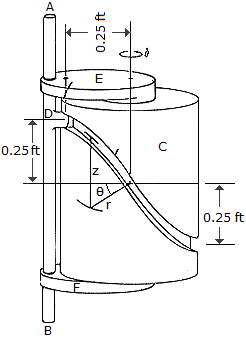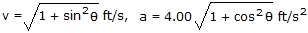# Engineering Mechanics - Kinematics of Particle (KOP) - Discussion

### Discussion :: Kinematics of Particle (KOP) - General Questions (Q.No.3)

3.The cylindrical cam C is held fixed while the rod AB and bearings E and F rotate about the vertical axis of the cam at a constant rate of= 4 rad/s. If the rod is free to slide through the bearings, determine the magnitudes of the velocity and acceleration of the guide D on the rod as a function of. The guide follows the groove in the cam, and the groove is defined by the equations r = 0.25 ft and z = (0.25 cos) ft.

 [A].[B]. v = cosft/s, a = 4.00 sinft/s2 [C]. v = sinft/s, a = 4.00 cosft/s2 [D]. v = 1.000 ft/s, a = 4.00 ft/s2

Explanation:

No answer description available for this question.

 Jim said: (Feb 19, 2017) How to solve this? Please tell me.